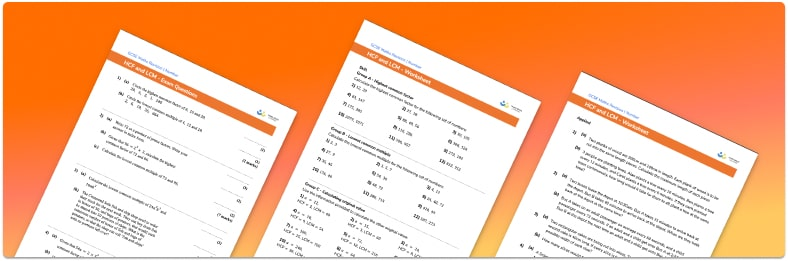# Highest Common Factor And Lowest Common Multiple WorksheetHelp your students prepare for their Maths GCSE with this free Highest Common Factor and Lowest Common Multiple worksheet of 44 questions and answers

• Section 1 of the HCF and LCM Worksheet contains 36 skills-based questions, in 3 groups to support differentiation
• Section 2 contains 4 applied Highest Common Factor and Lowest Common Multiple questions with a mix of worded problems and deeper problem solving questions
• Section 3 contains 4 foundation and higher level HCF and LCM GCSE exam style questions
• Answers and a mark scheme for all Highest Common Factor and Lowest Common Multiple questions
• Follows variation theory with plenty of opportunities for students to work independently at their own level
• All questions created by fully qualified expert secondary maths teachers
• Suitable for GCSE maths revision for AQA, OCR and Edexcel exam boards

• This field is for validation purposes and should be left unchanged.

You can unsubscribe at any time (each email we send will contain an easy way to unsubscribe). To find out more about how we use your data, see our privacy policy.

### Highest common factor and lowest common multiple at a glance

The highest common factor (HCF/greatest common factor/GCF) of two or more numbers is the biggest number which is a factor of each number. To find HCF of two numbers we can either write out all the factors of each number and select the highest number which appears in both lists or use the prime factorisations of the numbers.

The lowest common multiple (LCM/least common multiple) of two or more whole numbers is the smallest number which is a multiple of each number. To find the lowest common multiple of two numbers we can either write out the multiples of each given number until we identify one which is the same or use the prime factors of the numbers.

Highest common factors and lowest common multiples are useful in other areas of maths. For example, we use the highest common factor when factorising in algebra and we use the lowest common multiple when adding and subtracting fractions to find the lowest common denominator.

Looking forward, students can then progress to additional number worksheets, for example aorFor more teaching and learning support on Number our GCSE maths lessons provide step by step support for all GCSE maths concepts.

## Do you have KS4 students who need more focused attention to succeed at GCSE?There will be students in your class who require individual attention to help them succeed in their maths GCSEs. In a class of 30, it’s not always easy to provide.

Help your students feel confident with exam-style questions and the strategies they’ll need to answer them correctly with our dedicated GCSE maths revision programme.

Lessons are selected to provide support where each student needs it most, and specially-trained GCSE maths tutors adapt the pitch and pace of each lesson. This ensures a personalised revision programme that raises grades and boosts confidence.

Find out more